• 多元函数求偏导问题是多元函数微分学中的一项重点和难点内容。在求解这类题目时, 既要严 格区分自变量与中间变量, 而且要注意不能丢掉偏导函数作为复合函数时的偏导数
• 本博文源于matlab求解多元函数导数。涉及求一阶/求多阶/求向量偏导数/求隐函数导数
本博文源于matlab求解多元函数导数。涉及求一阶/求多阶/求向量偏导数/求隐函数导数
多元函数的偏导数
diff(f(x,y,z),变量名)

例子 求x^2+lny+根号z的偏导数>> syms x
>> syms y
>> syms z
>> du_dx = diff(x^2+log(y)+sqrt(z),x)

du_dx =

2*x

>> du_dz = diff(x^2+log(y)+sqrt(z),z)

du_dz =

1/2/z^(1/2)

>>

求高阶偏导数
diff(f(x,y,z),变量名,n)

例子：将上面的函数求4阶
>> du_dz = diff(x^2+log(y)+sqrt(z),z,4)

du_dz =

-15/16/z^(7/2)


求多元向量函数的偏导数
利用Jacobian矩阵求解
jacobian([f,g,h],[x,y,z]).

例子：求u的向量偏导数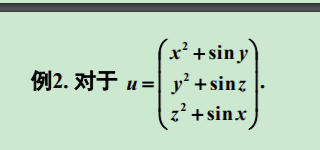syms x y z;
>> jacobian([x^2+sin(y),y^2+sin(z),z^2+sin(x)],[x,y,z])

ans =

[    2*x, cos(y),      0]
[      0,    2*y, cos(z)]
[ cos(x),      0,    2*z]

>>

求隐函数形式偏导数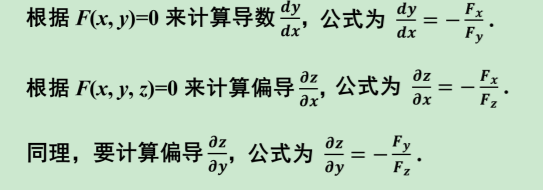例子1：求dy/dz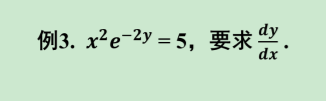>> syms x y
>> F=x^2*exp(-2*y)-5;
>> dy_dx=-diff(F,x)/diff(F,y)

dy_dx =

1/x

>>

例子：求偏导数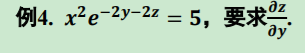>> syms x y
>> F=x^2*exp(-2*y-2*z)-5;
>> dz_dy=-diff(F,y)/diff(F,z)

dz_dy =

-1

>>



展开全文matlab 数学建模
• 以二元函数的二阶偏导数为例，偏x导数两个偏导数、偏y导数两个偏导数。 定理：如果二元函数的两个二阶混合偏导数连续，那么他们两个相等。 全微分 与一元函数类似，由于两个变量，x或y的增量称为偏增量，单单...
偏导数全导数
偏导数
由于是二元函数，有两个因变量。偏导数表示分别对某一个导数求导，如偏x导数、偏y导数。
高阶偏导数
对偏导数继续求导。以二元函数的二阶偏导数为例，偏x导数有两个偏导数、偏y导数有两个偏导数。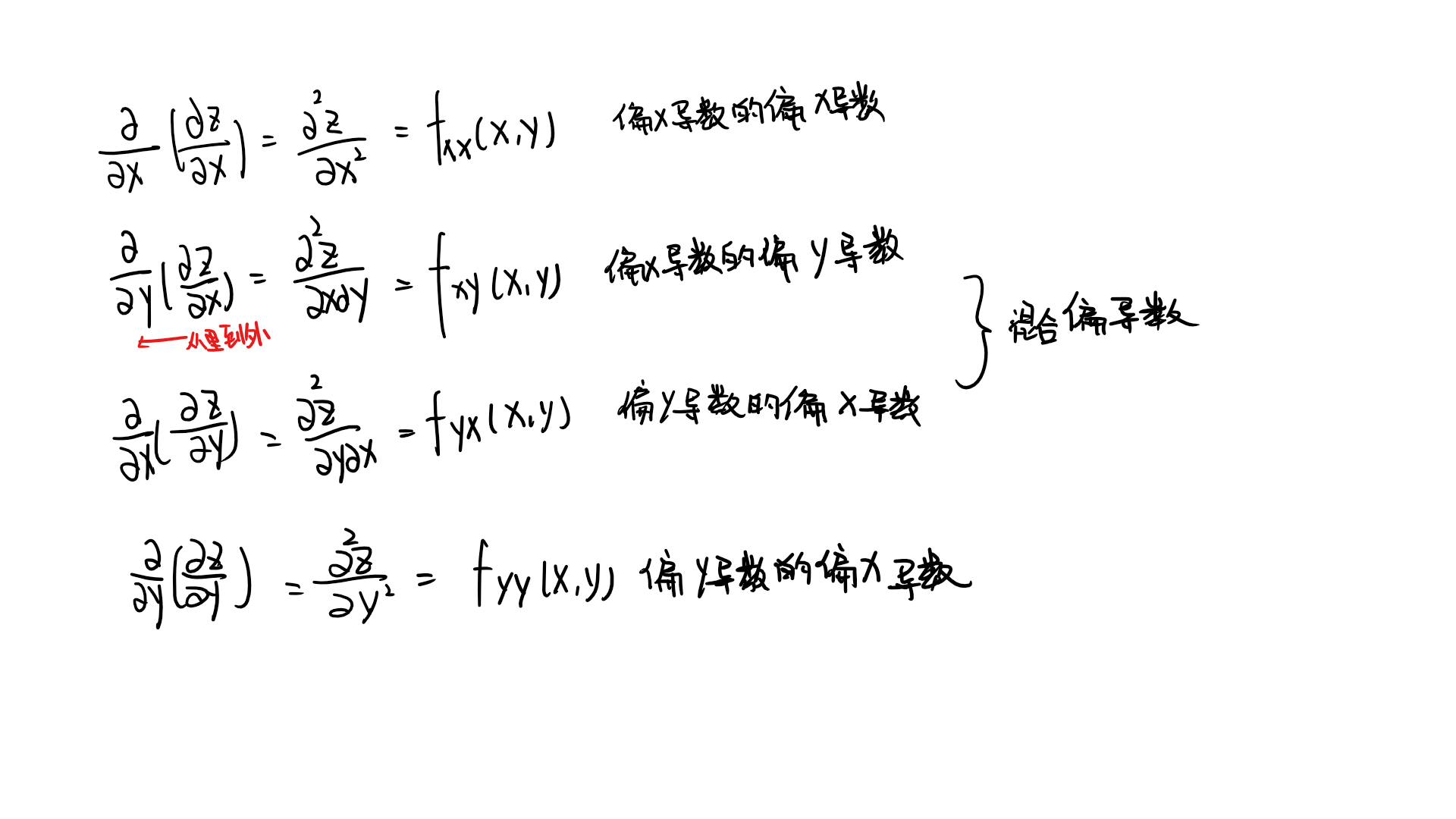定理：如果二元函数的两个二阶混合偏导数连续，那么他们两个相等。
全微分
与一元函数类似，由于有两个变量，x或y的增量称为偏增量，单单对x或y的微分称为偏微分。
若x，y同时增加，称为全增量。
全微分定义见下图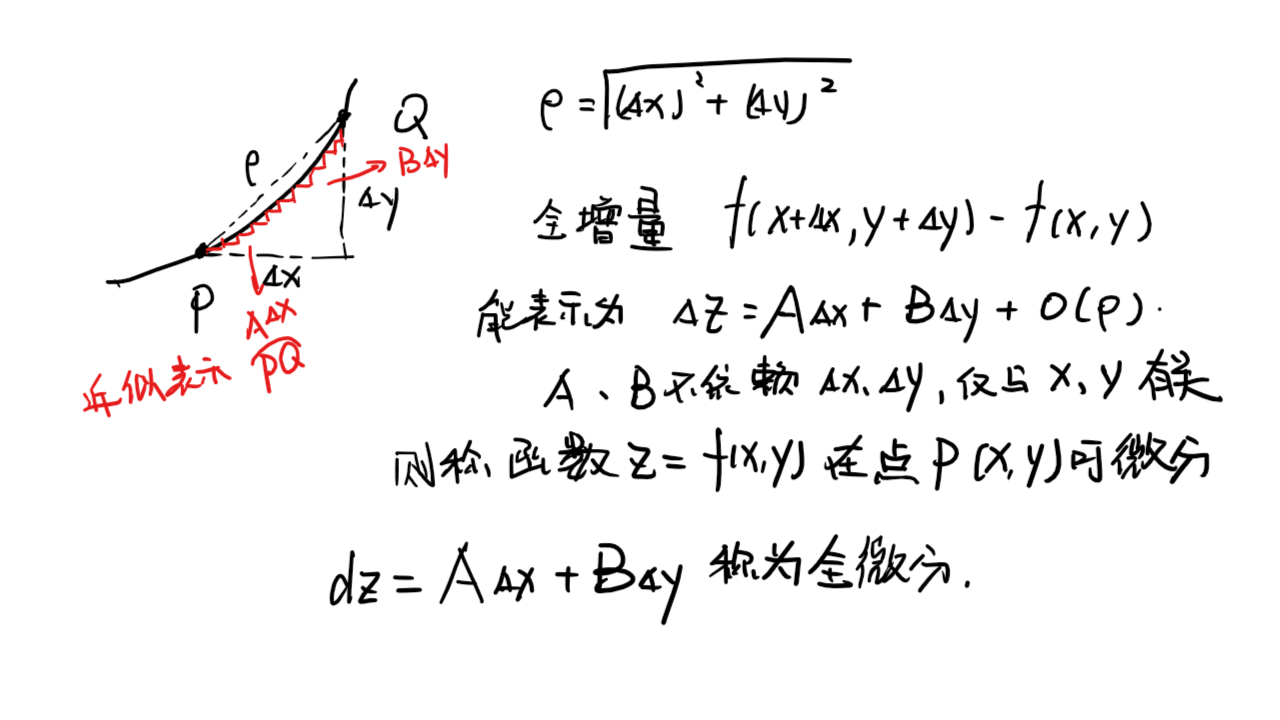定理

如果函数在该点可微分，那么其在该点的偏导数一定存在，且全微分中A、B分别等于偏x导数、偏y导数（叠加定理）
（全微分存在，函数可微分，偏导数一定存在；偏导数存在，全微分不一定存在）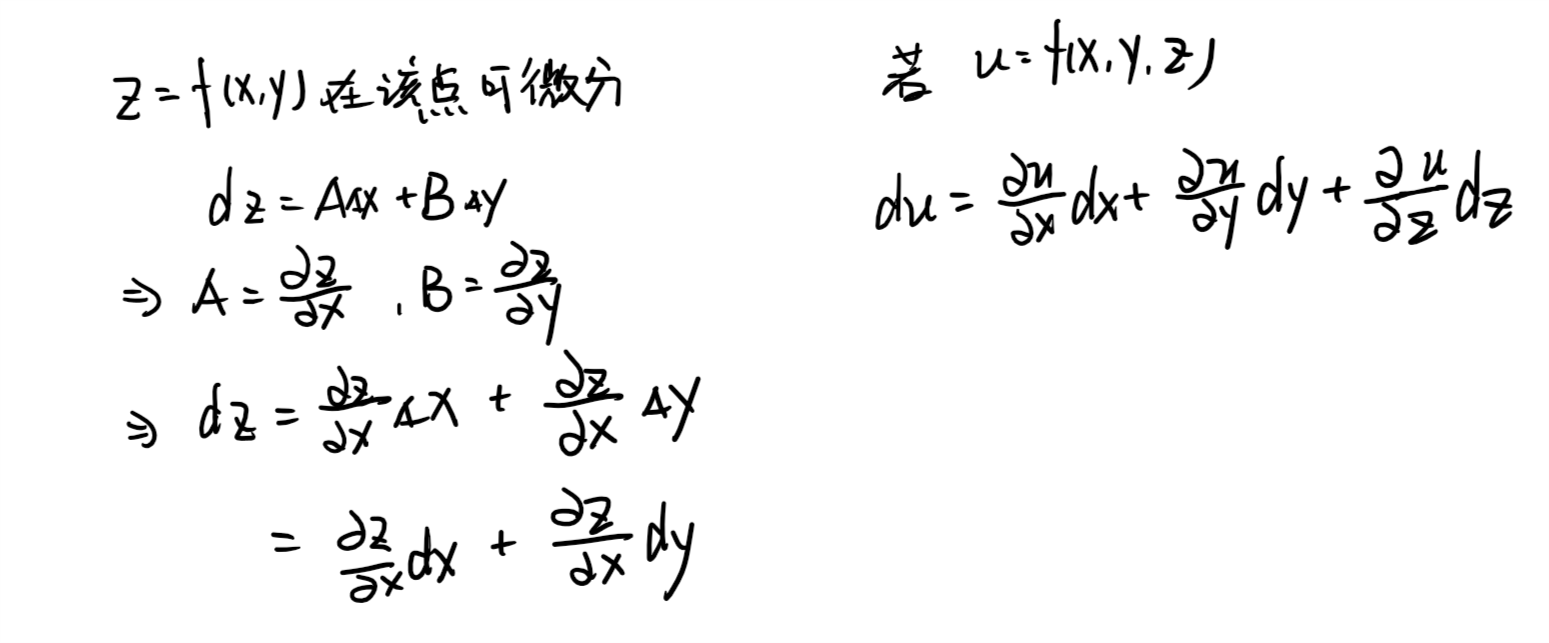如果函数在该点偏导数连续，那么函数在该点可微分

多元复合函数求导
一元函数与多元函数复合
先对多元函数微分，再把每个函数看成一元函数进行求导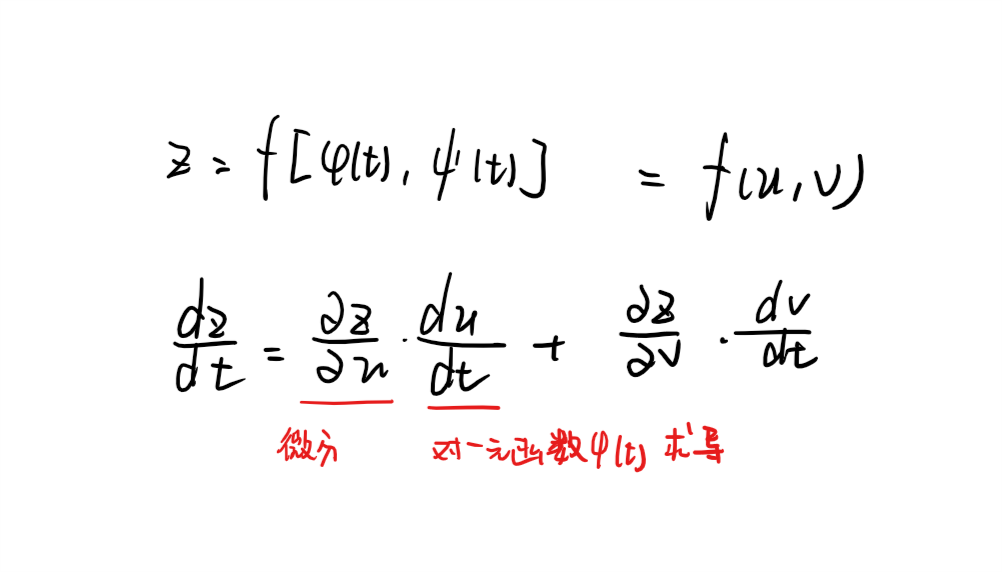多元函数与多元函数复合
如果对x求导，就先对所有函数微分，再把每个函数对x微分，最后相加。对y同理。其他情形
当多元函数与一元或者多元函数复合时，可能所导变量在某个函数中不存在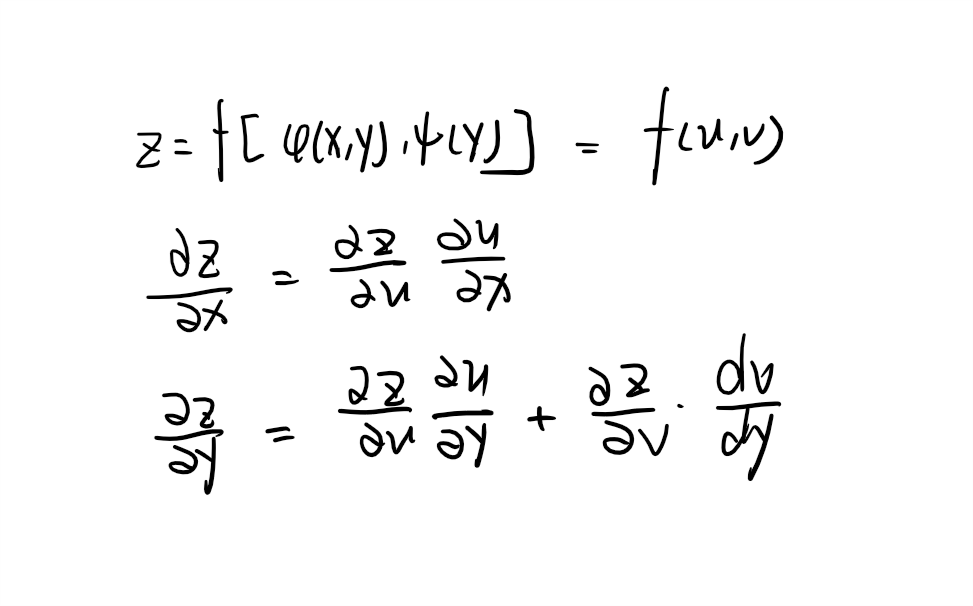不管那种情况，都有一下规律：
把最外层函数里的一个一个函数看过来，如果这个函数不存在所导变量，就不理他看下一个（微分后为0）。如果有，就先把最外层函数对其微分，如果里面这个函数是一元函数，就对变量求导；如果是多元，就对变量微分。
多元函数二阶求导
为方便起见，做出如下定义：有z=f(u,v)。f1’(u,v)=fu(u,v)——f对u求偏导;f2’=fv(u,v)——f对v求偏导;f12’’(u,v)=fuv(u,v)等等…
先求一阶偏导，再根据公式求二阶偏导数。需要注意的是此处求出来的是一阶偏导对变量的微分。由于一阶偏导内涵中间变量u、v，因此要再进行微分将一阶偏导对变量的微分变成二阶偏导。
隐函数求导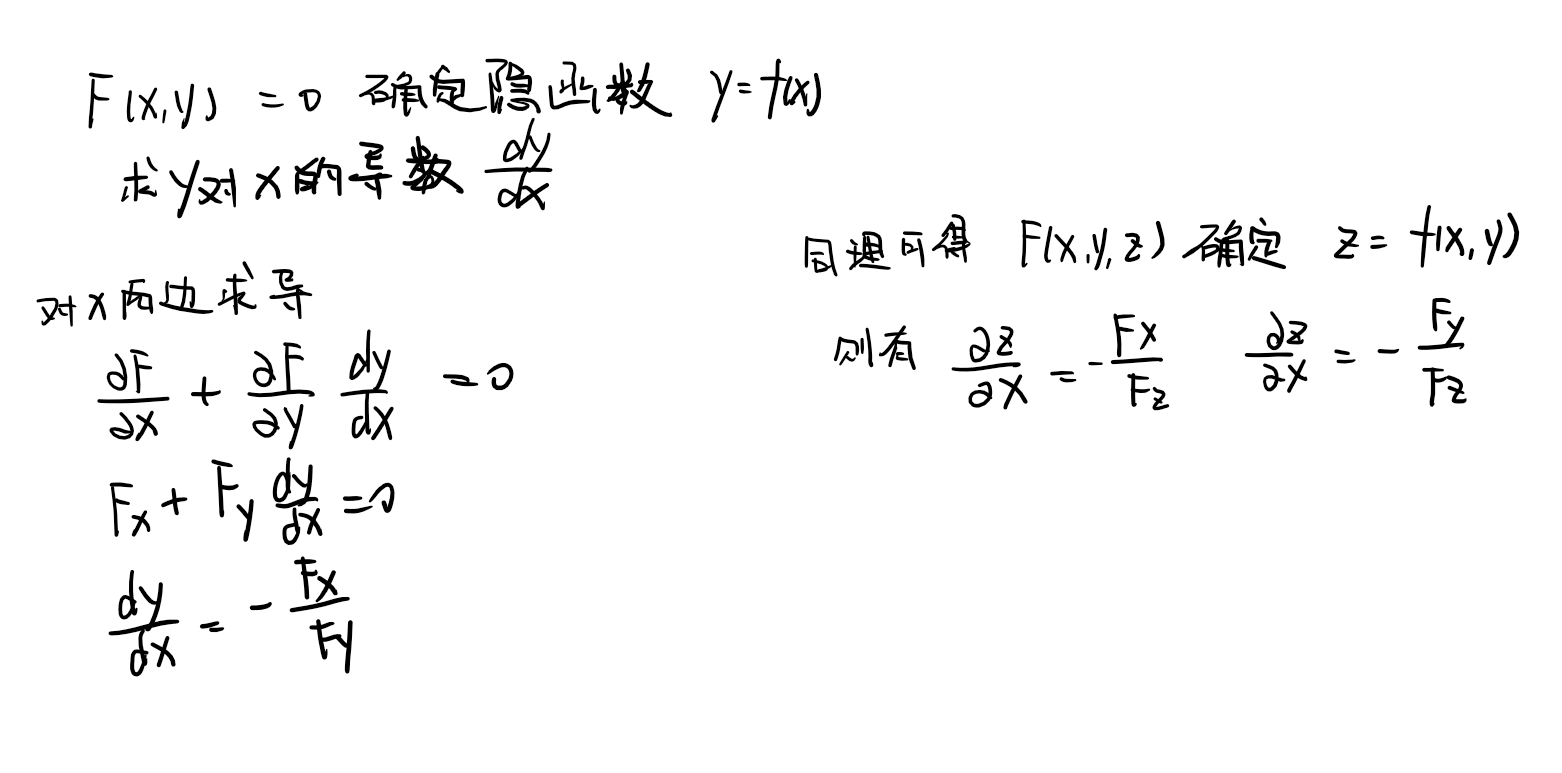方程组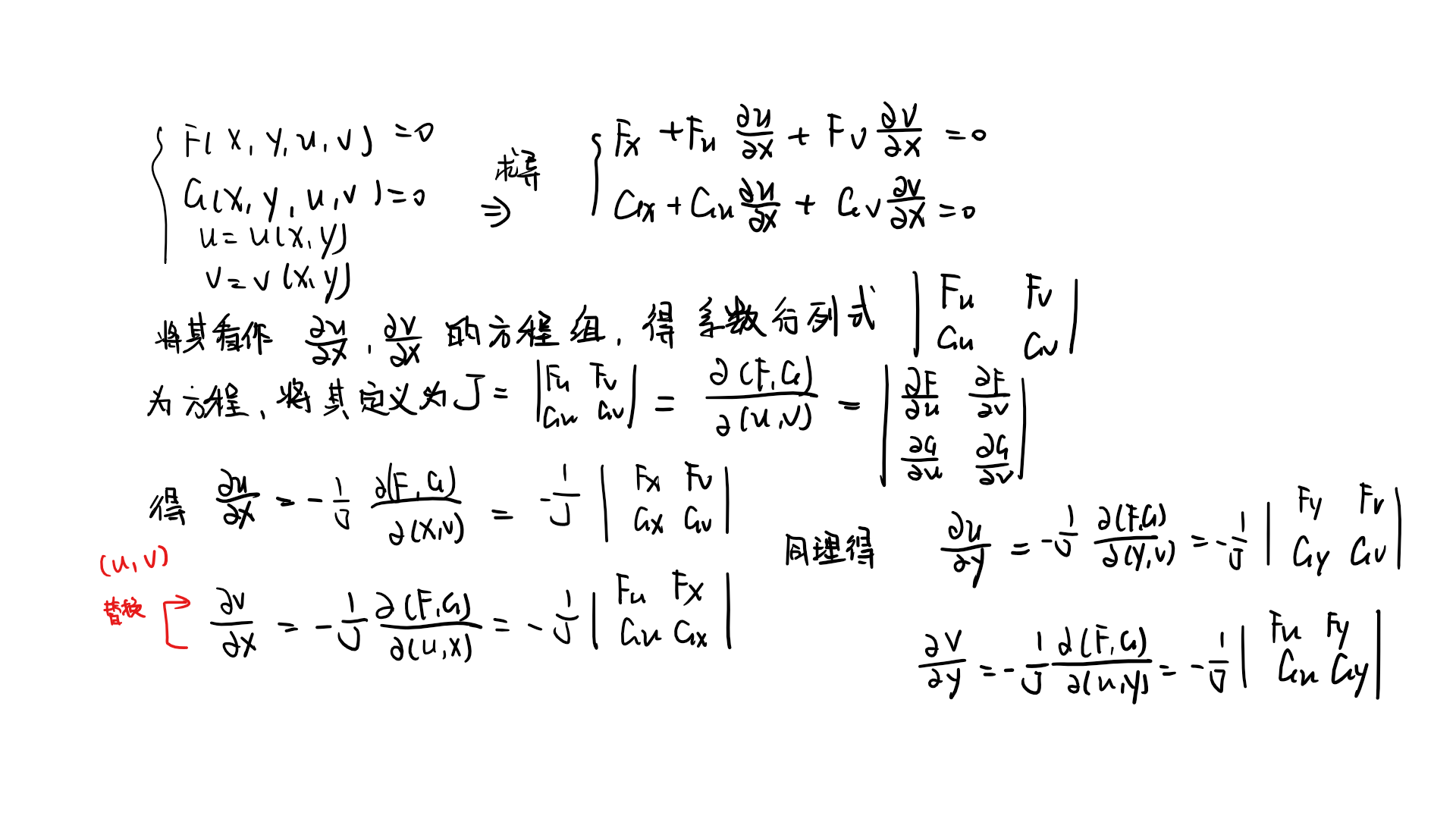在求解的时候可以把行列式右边的常数和所求的变量前的系数代换，利用行列式法则求解。
以下给出例题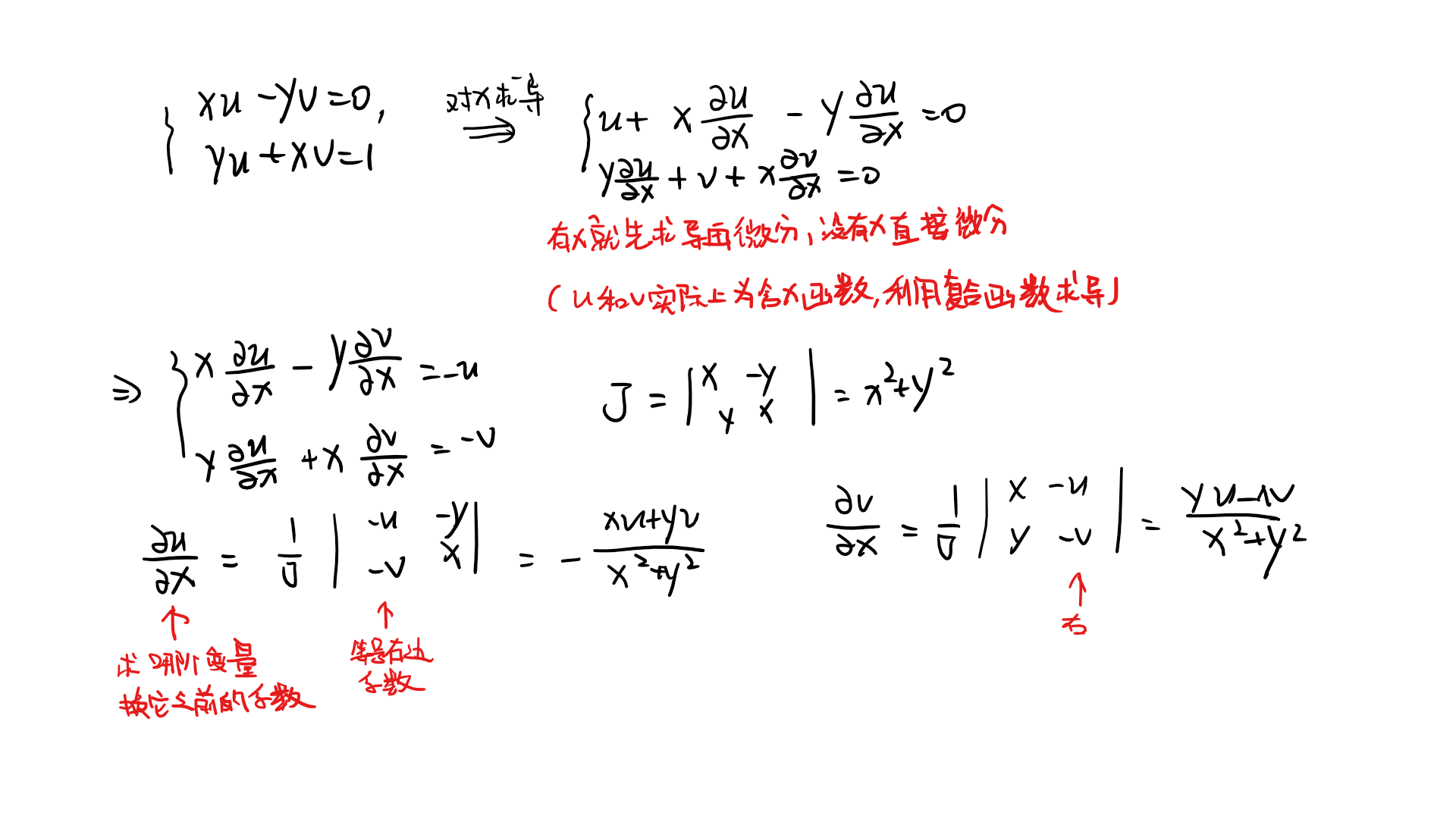展开全文高等数学 全导数 隐函数
• 偏导数 ...对多元函数而言，即使函数的各个偏导数都存在，也不能保证函数在该点连续。 即是可导不一定连续 3.高级偏导数 四个二阶偏导数 其中二三叫做混合偏导数 二阶及二阶以上的叫做高阶偏导数 ...
偏导数

注意：偏导数的表示是一个整体
并且这种情况只能用定义来做

1.偏导数的概念
2.偏导数的几何意义
对多元函数而言，即使函数的各个偏导数都存在，也不能保证函数在该点连续。
即是可导不一定连续
3.高级偏导数
四个二阶偏导数
其中二三叫做混合偏导数
二阶及二阶以上的叫做高阶偏导数
一元复合函数推广
1.复合函数的求导法则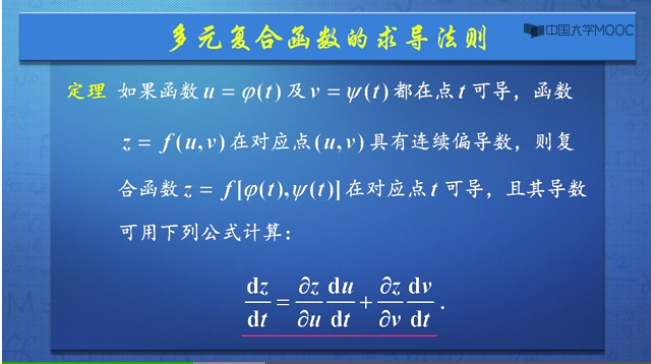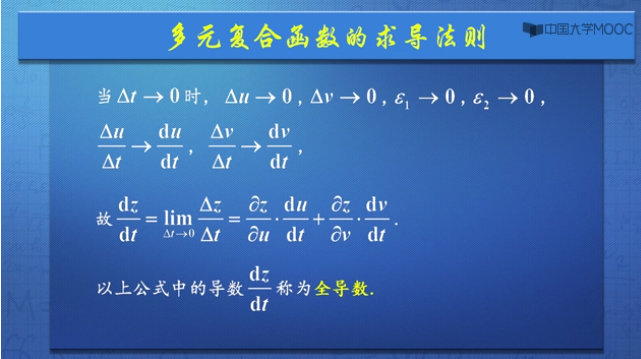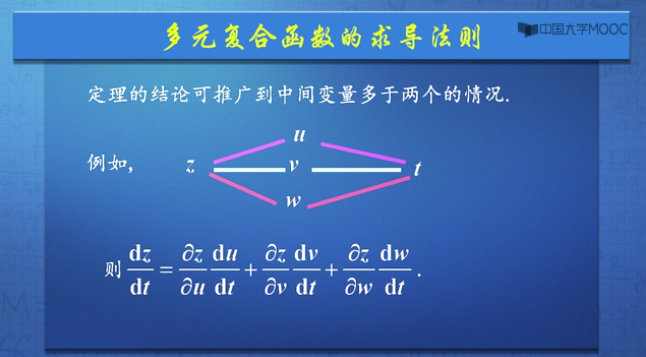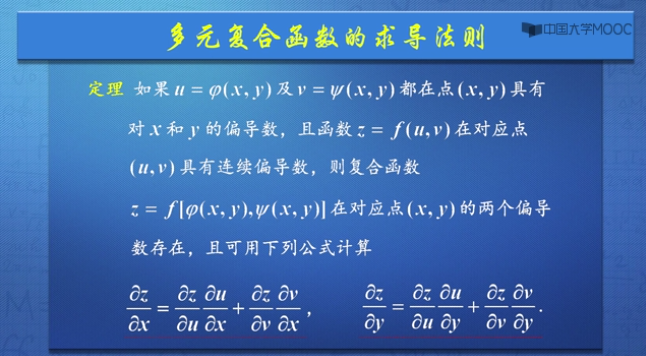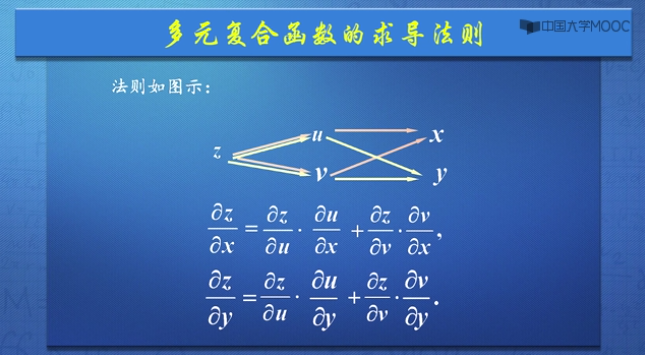推广：对于多元函数自变量大于两个的时候也成立。
2.求导法则的应用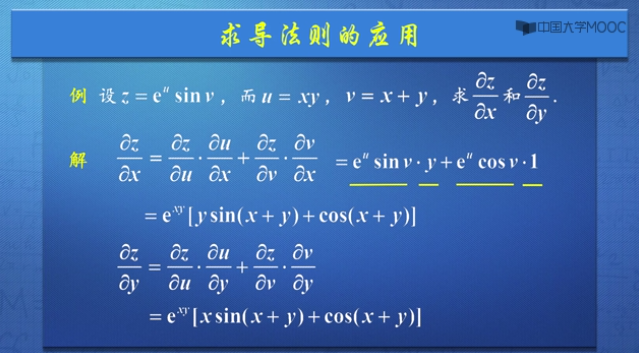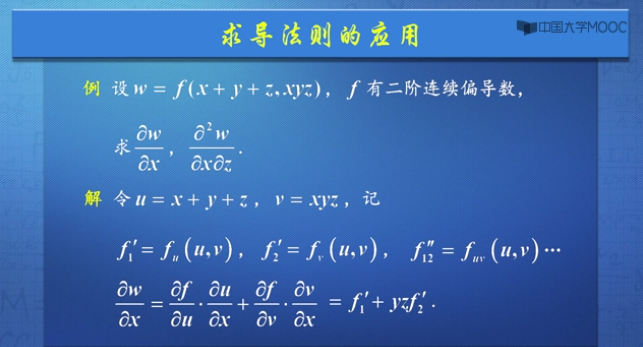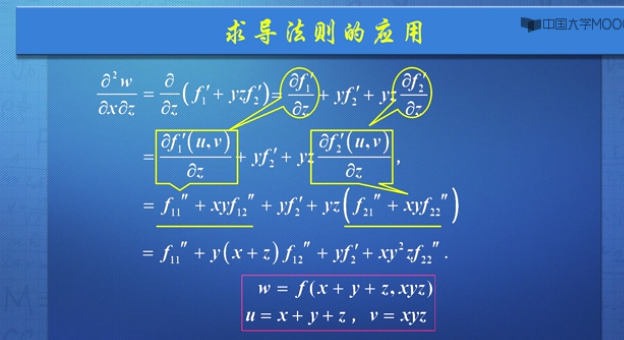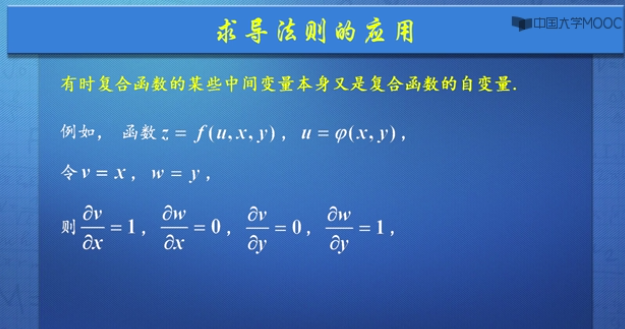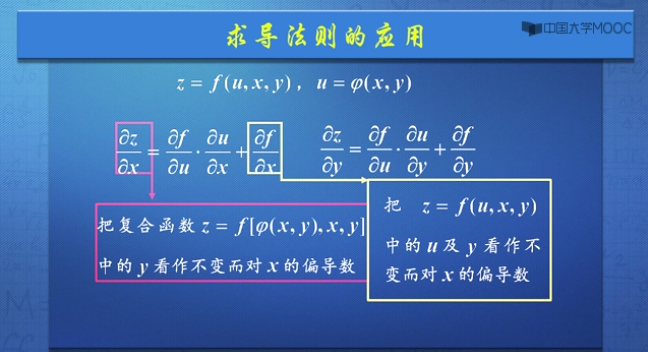3.全微分形式不变性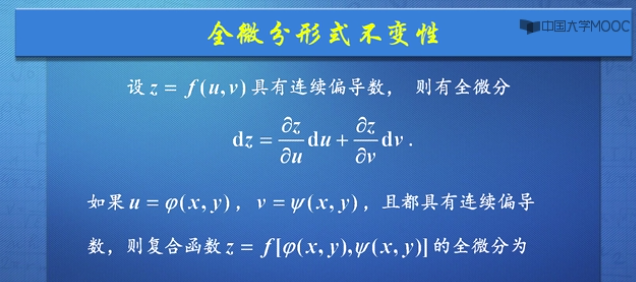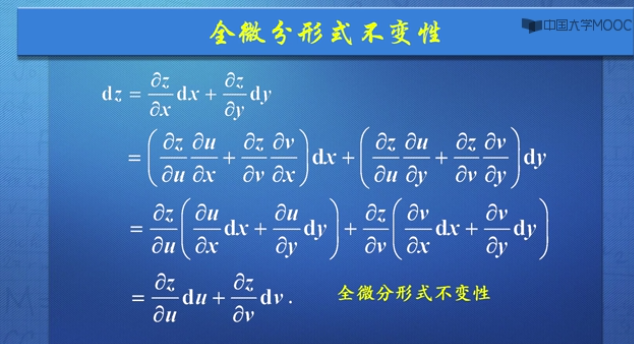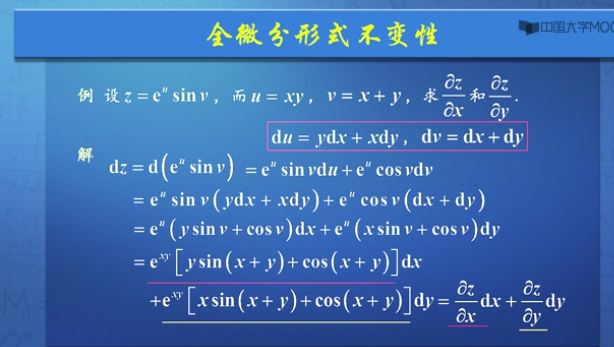注意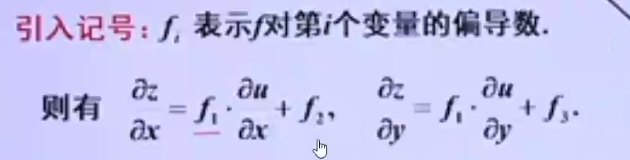展开全文• 1.函数：从一元到多元 在前面的两讲内容中，我们所介绍的函数都只有一个自变量，而从这一讲开始，我们关心和感兴趣的是含多个实数自变量的实值函数。例如，对于二元函数而言，就是在某平面集合DDD内任给有序变量(x,y...
1.函数：从一元到多元
在前面的两讲内容中，我们所介绍的函数都只有一个自变量，而从这一讲开始，我们关心和感兴趣的是含多个实数自变量的实值函数。例如，对于二元函数而言，就是在某平面集合$D$内任给有序变量$(x,y)$，能够确定唯一的实数$f(x,y)$，例如：
$f(x,y)=\sqrt{x^2+y^2}$
$f(x,y)=y^2-x^2$
一般我们也将其写作：$z=\sqrt{x^2+y^2}$，以及$z=y^2-x^2$。因此我们称$x$，$y$为函数的自变量，$z$为函数的因变量。
2.二元函数的可视化
2.1.函数图像的绘制
下面，我们来实践一下如何利用$python$来绘制三维曲面：$z=x-\frac{1}{9}x^3-\frac{1}{2}y^2$。
代码片段：
from matplotlib import pyplot as plt
import numpy as np
from mpl_toolkits.mplot3d import Axes3D

fig = plt.figure()
ax = Axes3D(fig)

x = np.arange(-4, 4, 0.01)
y = np.arange(-4, 4, 0.01)
x, y = np.meshgrid(x, y)
z = x-(1./9)*x**3-(1./2)*y**2

ax.plot_surface(x, y, z, cmap=plt.cm.Blues_r)
plt.show()

运行结果：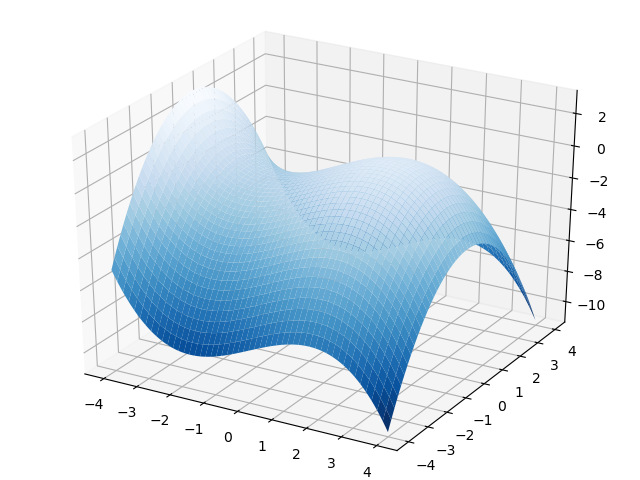2.2.等高线图的绘制
在三维图形的绘图中，还有一种呈现三维图形性质的表示方法，那就是等高线图。等高线图大家不会陌生，他的定义是，利用每一个水平面$z=c$与函数图形表面相交于一条曲线，然后将所有的这些曲线投影到$xoy$平面上，就得到不同高度（也就是$z=f(x,y)$）取值的等高线集合，即构成了这个三维图形的等高线图。
我们下面接着来绘制上面这幅三维图像的等高线图：
代码片段：
import numpy as np
import matplotlib.pyplot as plt

def f(x, y):
return x-(1./9)*x**3-(1./2)*y**2

x = np.arange(-4, 4, 0.01)
y = np.arange(-4, 4, 0.01)

#把x,y数据生成mesh网格状的数据
X, Y = np.meshgrid(x, y)

#填充等高线间的颜色
plt.contourf(X, Y, f(X, Y), 24, cmap=plt.cm.hot)
#添加等高线
C = plt.contour(X, Y, f(X, Y), 24)
#增加各等高线的高度值
plt.clabel(C, inline=True, fontsize=12)

plt.show()

运行结果：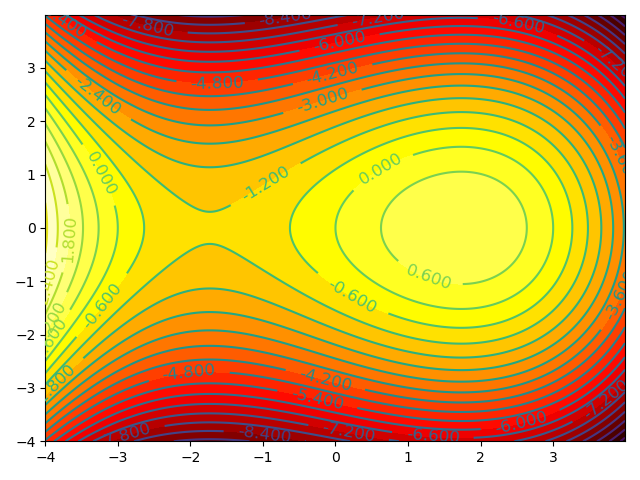我们这里简单的解释一下这段代码：
在函数$plt.contour$当中，$24$表示的是我们使用的等高线的数量，数字越大，等高线越多
通过函数$plt.contourf$，在等高线之间填充不同的颜色以示区分，其中，$cmap=plt.cm.hot$表示我们的等高线集合使用热力图的颜色集，颜色越浅表示函数值越大，就和火焰温度和火焰颜色的关系一样。
3.多元函数的偏导数
3.1.概念的引入
我们在前面已经介绍过一元函数的导数，那么从一元变量过渡到多元变量之后，导数又该如何定义？
假如函数$f(x,y)$是关于$x$和$y$两个变量的函数，我们在讨论导数概念的时候需要固定一个变量再去讨论另外一个变量。例如，我们需要先将$y$固定为一个常数$y=y_0$，此时$f(x,y_0)$实际上就变成了一个只有单个变量$x$的函数，那么他在$x=x_0$处的导数称为是$f$关于$x$在$(x_0,y_0)$处的偏导数，被记作是：$f_x(x_0,y_0)$。
那么按照我们之前利用极限来定义导数的式子进行迁移拓展，我们很容易得到偏导数的定义式，即：$f_x(x_0,y_0)=lim_{\Delta x\rightarrow 0}\frac{f(x_0+\Delta x,y_0)-f(x_0,y_0)}{\Delta x}$
那么同样的，函数$f(x,y)$关于$y$在$(x_0,y_0)$处的偏导数，记作是：
$f_y(x_0,y_0)=lim_{\Delta y\rightarrow 0}\frac{f(x_0,y_0+\Delta y)-f(x_0,y_0)}{\Delta y}$
和导数中的线性算子$\frac{d}{dx}$类似，偏导数中也有自己的特色符号$\partial$，那么$\frac{\partial}{\partial x}$和$\frac{\partial}{\partial y}$则分别表示对$x$和$y$求偏导的线性算子。
那么，针对多元函数$z=f(x,y)$，下面的这一系列描述都是等效的：$f_x(x,y)=\frac{\partial z}{\partial x}=\frac{\partial f(x,y)}{\partial x}$。
针对$(x_0,y_0)$的具体取值$f_x(x_0,y_0)$，则可以记作是$f_x(x_0,y_0)=\frac{\partial z}{\partial x}|_{(x_0,y_0)}$
关于$y$的偏导数也是同理，这里就不再赘述了。
3.2.偏导数的几何意义
下面我们来探讨一下偏导数的几何意义。我们还是来看$z=x-\frac{1}{9}x^3-\frac{1}{2}y^2$这个二元函数，来研究一下$\frac{\partial z}{\partial x}$。请大家记住，在求关于变量$x$的偏导数时，$y$就是一个与变量$x$无关的常数。
结合下面的代码和示意图来看$f_x(x_0,y_0)$的几何含义。在代码例子中，我们实际举例$x_0=3,y_0=-2$，首先，我们让$y=-2$这个橙色的垂直平面与整个蓝色曲面相交，形成曲面表面那条红色的相交曲线，也就是说这条红色曲线既在表示原函数的蓝色曲面上，也在平行于$xoz$平面的橙色平面$y=-2$上。
我们过点$(x_0,y_0,f(x_0,y_0))$，在图中就是那个位于$(3,-2,-2)$的黑色点，做红色曲线的切线。那么最终原函数$z$关于$x$的偏导数的取值：$f_x(3,-2)$就是这条切线的斜率，需要注意的是，这条切线同样也在垂直平面$y=-2$中，与$xoz$平面平行。
代码片段：
from matplotlib import pyplot as plt
import numpy as np
from mpl_toolkits.mplot3d import Axes3D

fig = plt.figure()
ax = Axes3D(fig)

#多元函数z=f(x,y)
def f(x, y):
return x-(1./9)*x**3-(1./2)*y**2

#绘制原函数的图像
x = np.arange(-4, 4, 0.01)
y = np.arange(-4, 4, 0.01)
x, y = np.meshgrid(x, y)
ax.plot_surface(x, y, f(x, y), alpha=0.7)

#绘制平面y=-2与
x = np.arange(-4, 4, 0.01)
z = np.arange(-8, 5, 0.01)
x, z = np.meshgrid(x, z)
y = x*z*0-2
ax.plot_surface(x, y, z, alpha=0.2)

#当确定y0=-2时，函数变成了一条平行于xoz平面的曲线
x = np.arange(-4, 4, 0.01)
y = np.array([-2]*len(x))
ax.plot(x, y, f(x, y), color='r')

#过(3,-2,f(3,-2))，做上述曲线的切线
#y=-2时，关于x的偏导数的方程为：z=-2x+4
ax.scatter(3, -2, f(3,-2), color='k')

x = np.arange(-1, 6, 0.01)
y = np.array([-2]*len(x))
z = -2*x+4
ax.plot(x, y, z, color='k')

plt.show()

运行结果：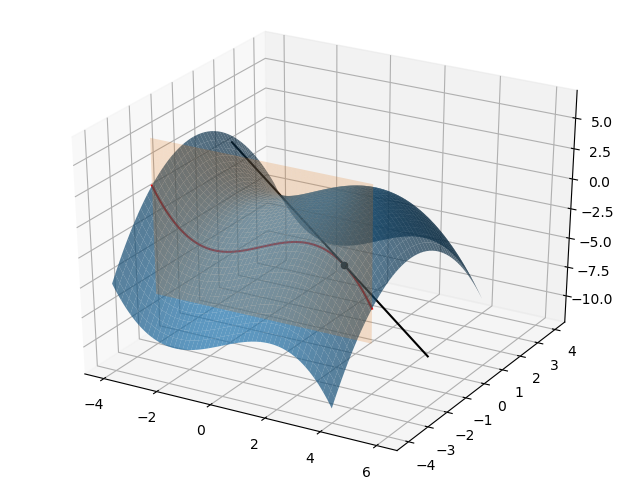3.3.高阶偏导数
我们知道，对于多元函数$f(x,y)$，无论是关于$x$的偏导数$f_x$：$\frac{\partial f}{\partial x}$，还是关于$y$的偏导数$f_y$：$\frac{\partial f}{\partial y}$，实际上仍然也都是函数，是关于变量$x$和$y$的函数。
因此这些偏导函数再对变量$x$和$y$进一步求偏导，就能够得到阶数更高的偏导数，也就是函数$f$的二阶偏导数，显然二元函数$f(x,y)$的二阶偏导数有四个：
$f_{xx}=\frac{\partial}{\partial x}(\frac{\partial f}{\partial x})=\frac{\partial^2 f}{\partial x^2}$
$f_{yy}=\frac{\partial}{\partial y}(\frac{\partial f}{\partial y})=\frac{\partial^2 f}{\partial y^2}$
$f_{xy}=\frac{\partial}{\partial y}(\frac{\partial f}{\partial x})=\frac{\partial^2 f}{\partial y \partial x}$
$f_{yx}=\frac{\partial}{\partial x}(\frac{\partial f}{\partial y})=\frac{\partial^2 f}{\partial x \partial y}$
因此，也请大家不要畏惧二阶偏导数，觉得他是一个新的概念，其实我们把他拆解开，先求函数$f(x,y)$的偏导数，在此基础上再求一次偏导数就好了，我们看一个实际的例子，大家就能弄明白：
我们对函数$f(x,y)=xe^y-sin(\frac{x}{y})+x^3y^2$求所有的二阶偏导数：
首先，我们先求函数关于变量$x$和变量$y$的偏导数：
$f_x(x,y)=e^y-\frac{1}{y}cos(\frac{x}{y})+3x^2y^2$
$f_y(x,y)=xe^y+\frac{x}{y^2}cos(\frac{x}{y})+2x^3y$
然后，我们在这两个一阶偏导数的基础上，求得所有四个二阶偏导数：
$f_{xx}(x,y)=\frac{1}{y^2}sin(\frac{x}{y})+6xy^2$
$f_{yy}(x,y)=xe^y+\frac{x^2}{y^4}sin(\frac{x}{y})-\frac{2x}{y^3}cos(\frac{x}{y})+2x^3$
$f_{xy}(x,y)=e^y-\frac{x}{y^3}sin(\frac{x}{y})+\frac{1}{y^2}cos(\frac{x}{y})+6x^2y$
$f_{yx}(x,y)=e^y-\frac{x}{y^3}sin(\frac{x}{y})+\frac{1}{y^2}cos(\frac{x}{y})+6x^2y$
4.多元函数的极限与连续
4.1.多元函数极限的概念
和一元函数相比，多元函数极限的概念要相对麻烦一点：$lim_{(x,y)\rightarrow (a,b)}f(x,y)=L$。
多元函数$f(x,y)$极限的定义是$f(x,y)$的值在$(x,y)$趋近于$(a,b)$时趋近于$L$，但是问题的关键是$(x,y)$趋近于$(a,b)$的方式，我们回顾一下一元函数$f(x)$在$c$点处存在极限的定义，他是要求函数的左极限等于右极限，即：$lim_{x\rightarrow c^{-}} f(x)=lim_{x\rightarrow c^{+}}f(x)$，要求函数的自变量从$x$轴的正负两个方向分别逼近取值点$c$。
那么拓展到二元函数$f(x,y)$，极限的定义也是一样，他需要在平面上满足$(x,y)$无论从哪个方向趋近于$(a,b)$都应该得到相同的$L$值，那么二元函数的极限$f(x,y)$就一定存在并且为$L$。
下面这个严格的定义就能够包含这层意思：
如果我们任意给出一个很小的值$\varepsilon>0$，都存在$\delta>0$，使得当$0<|(x,y)-(a,b)|<\delta$时，$|f(x,y)-L|<\varepsilon$成立。则称当$(a,b)$趋近于$(x,y)$时，$f(x,y)$的极限为$L$，我们将其记作是：$lim_{(x,y)\rightarrow (a,b)}f(x,y)=L$
这里和一元函数中的左右极限相对应的就是$0<|(x,y)-(a,b)|<\delta$，换个写法可能会更加清晰一些：$0<\sqrt{(x-a)^2+(y-b)^2}<\delta$，这是一个计算点$(x,y)$和$(a,b)$的距离公式，他表明$(x,y)$的取值范围是以$(a,b)$为圆心，$\delta$为半径的圆。这就实现了$(x,y)$在二维平面内以任意路径逼近$(a,b)$时，函数$f(x,y)$的取值都能任意接近$L$的效果。
因此结合上面的定义，同时回顾一元函数$f(x)$的极限定义，我们抓住两点核心即可：
第一点就是$(x,y)$逼近$(a,b)$的路径是任意的，如果通过不同的路径方式逼近$(a,b)$得到了不同的值，那么这个多元函数在$(a,b)$处的极限就是不存在的。
第二点就是函数$f(x,y)$在$(a,b)$处有极限$L$，可以允许函数在$(a,b)$处的取值$f(a,b) \neq L$，甚至没有定义，这一点大家类比一下一元函数极限的内容就很清楚了。
回过头来我们会发现，二元函数极限的概念和一元函数的核心本质是一样的，因此将极限的定义拓展到三元乃至$n$元函数我们也不会感到陌生。
4.2.多元函数的连续性
我们还是以二元函数为例，首先抛出满足连续性的条件定义，那就是：
$lim_{(x,y)\rightarrow (a,b)}f(x,y)=f(a,b)$
结合前面的内容和一元函数连续性的定义，这里面包含了两层的关键含义：
首先就是函数$f$在$(a,b)$处的极限要存在。
其次函数$f$在$(a,b)$处要有定义，并且其取值要与极限值相等。
那么在单点的连续性上，进一步讨论区域上的连续性就不那么难了，显然，若函数$f(x,y)$在区域$S$中的每一点都连续，那么我们就称函数$f(x,y)$在区域$S$内连续。
4.3. 观察：二阶偏导数的对称性
这里回过头来，我们不要放过前面的一个小小细节，在我们举的$f(x,y)=xe^y-sin(\frac{x}{y})+x^3y^2$的例子中，我们发现
$f_{xy}(x,y)=e^y-\frac{x}{y^3}sin(\frac{x}{y})+\frac{1}{y^2}cos(\frac{x}{y})+6x^2y$
$f_{yx}(x,y)=e^y-\frac{x}{y^3}sin(\frac{x}{y})+\frac{1}{y^2}cos(\frac{x}{y})+6x^2y$
$f_{xy}=f_{yx}$，这是偶然吗？其实不是，这里我们给出一个结论：那就是如果在区域$S$中$f_{xy}$和$f_{yx}$分别连续，那么在区域$S$内的每一点都满足$f_{xy}=f_{yx}$，换句话说就是函数改变混合偏导数的求导顺序，最终的结果是不变的。这个结论非常重要，后面在黑塞矩阵中我们还会见到。


展开全文自然语言处理 神经网络 数据挖掘 深度学习 tensorflow
• 包括全微分、偏导数、方向导数、梯度、全导数等内容。 初学这些知识的时候，学生会明显觉得这些概念不难掌握，而且定义及计算公式也很容易记住，但总觉得差那么点东西，说又不知道从何说起。反正笔者是这种感觉。...
• 多元复合函数的偏导数问题引入多元复合函数的求导法则定理1（一个自变量的情形）定理2（两个自变量的情形）多元函数求导法则应用例1例2例3例4例5多元函数一阶微分形式不变性例6 问题引入 多元复合函数的求导法则 ...算法
• 实验九 用Mathematica 软件求函数偏导数多元函数的极值 实验目的: 掌握用Mathematica 软件求函数偏导数与全微分多元函数的极值的语句和 方法 实验过程与要求: 教师利用多媒体组织教学,边讲边操作示范 实验的内容: ...
• 偏导数的概念 本质上就是求一元函数的导，只不过是把其他变量看作常数就行了。 在图像上显示可以想象下，例如z=f(x，y)这是一个三维 图形，然后对x求偏导其实就这一点所在的平行于zx平面的切面是投影到z，x上的...
• 偏导数的存在只能保证与坐标轴平行的方向上函数的极限值等于函数值（仅仅是坐标轴平行的方向），但是连续是指函数以任何方向趋近于某一定点，二元函数本身是一个平面型的，趋于某一定点是从四面八方的，而平行于坐标...
• MATLAB中使用diff函数求一元函数的导数，多元函数偏导以及矩阵向量的差分matlab
• ## 为什么偏导数连续，函数就可微？

万次阅读 多人点赞 2018-10-23 17:50:27
如果函数 的偏导数 、 在点 连续，那么函数在该点可微。 下面来解释这个结论，并且减弱这个结论的条件。 先简单阐述下“连续”、“偏导数”、“可微”的意义，后面要用到。如果非常熟悉了，可以直接跳到最后...微积分 高等数学 多变量微积分
• 2. 多元复合函数的几种情形（一个自变量的情形；多个自变量的情形） 3. 多元复合函数求导数的链式法则（树形图方法：沿线相乘，分线相加）：一个自变量的情形 4. 两个自变量的情形 6. 其他情形 ...
• 由前面给出的算法，可以编写出如下函数来求解隐函数偏导数函数说明 function dy = impldiff(f, x, y, n) %impldiff %隐函数求导 % 调用格式： % f1 = impldiff(f, x, y, n) % 其中：f=f(x,y), n为导数阶次 % ...数学建模 matlab
• 结论（一元函数范畴内） 可导与连续的关系：可导必连续，连续不一定可导； 可微与连续的关系：可微与可导...很显然函数连续，可导，可微和偏导数连续的关系可以从图中看出 函数连续不一定的函数可微（例子：y=|x|...
• §8.1 多元函数的基本概念 本章将在一元函数微分学的基础上，讨论多元函数的微分法及其应用。讨论中，我们主要以二元函数为主，因为从一元函数到二元函数会产生许多新问题，而从二元函数到二元以上的函数则可以类...高等数学
• 偏导数partial derivative 利用Sympy库 SymPy是一个符号计算的Python库。它的目标是成为一个全功能的计算机代数系统，同时保持代码简洁、易于理解和扩展。它完全由Python写成，不依赖于外部库。 SymPy支持符号计算...python
• 本文全面叙述多元函数的连续性、偏导数、方向导数及可橄性之间的关系。并通过实例澄清一些模糊看法。
• 【微积分5多元函数微分学】第五章第二节 偏导数与全微分的计算第二节 偏导数与全微分的计算1. 内容要点1. 复合函数求导2. 隐函数求导2. 常考题型1. 求一点处的偏导数与全微分2. 求已经给出具体表达式的偏导数与全...数据结构 leetcode python 人工智能 lambda...